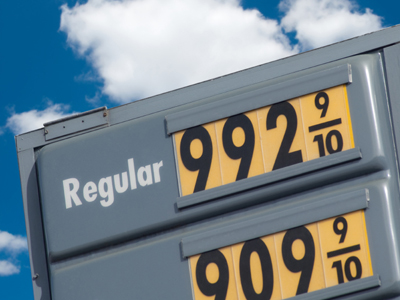992.9 is the same as 992910

# Place Value 4

This Math quiz is called 'Place Value 4' and it has been written by teachers to help you if you are studying the subject at elementary school. Playing educational quizzes is an enjoyable way to learn if you are in the 3rd, 4th or 5th grade - aged 8 to 11.

It costs only \$12.50 per month to play this quiz and over 3,500 others that help you with your school work. You can subscribe on the page at Join Us

We know the value of a digit changes with its position in a number relative to the decimal point. Before the decimal we have units, tens and hundreds, and after we have tenths, hundredths and thousandths. We also use place holders. Zeros at the end of a number are place holders. They come after another number, a 1 for example, to show whether it is worth 10, 100 or 1,000.

1.
Which is the number that is equivalent to one hundred and forty two point eight seven?
14287
142.87
1,428.7
142,087
142.87 has 1 hundred
4 tens
2 units
8 tenths
and 7 hundredths
2.
What needs to be added to 45.612 to make 45.682?
7
0.7
0.007
0.07
The 1 and the 8 are both in the hundredths column so 7100 or 0.07 must be added
3.
When we multiply a digit by ten what should we move?
We should move the digits one place to the left
We should move the digits one place to the right
We should move the digits ten places to the left
We should move the digits ten places to the right
So 300 x 10 = 3,000
4.
Which is the smallest of these numbers?
45 tenths
45 tens
45 hundredths
45 units
45 hundredths is equal to 0.45
5.
Which digit is a place holder?
0
1
2
9
0s at the start or end of a number are place holders
6.
What is the value of the digit 9 in 128.96?
9 hundredths
9 tenths
9 units
9 tens
The first column after the decimal point is tenths so the 9 = 910
7.
When 59,762 is divided by 10, what will we get?
59,7620
5,976.00
5,976.2
5,976,200
To divide by 10 move the decimal point one place to the left or the numbers one place to the right
8.
When we divide a number by one hundred what should we move?
We should move the digits one place to the left
We should move the digits one place to the right
We should move the digits two places to the left
We should move the digits two places to the right
So 300 divided by 100 = 3
9.
When 24,597 is multiplied by 100, what will we get?
245.97
245,970
2,459.7
2,459,700
To multiply by 100 move the decimal point two places to the right or the numbers two places to the left
10.
What does the digit 3 in 62.13 represent?
3 hundredths
3 tenths
3 units
3 tens
Numbers to the right of a decimal point represent 10ths 100ths 1,000ths 10,000ths etc...
Author:  Amanda Swift